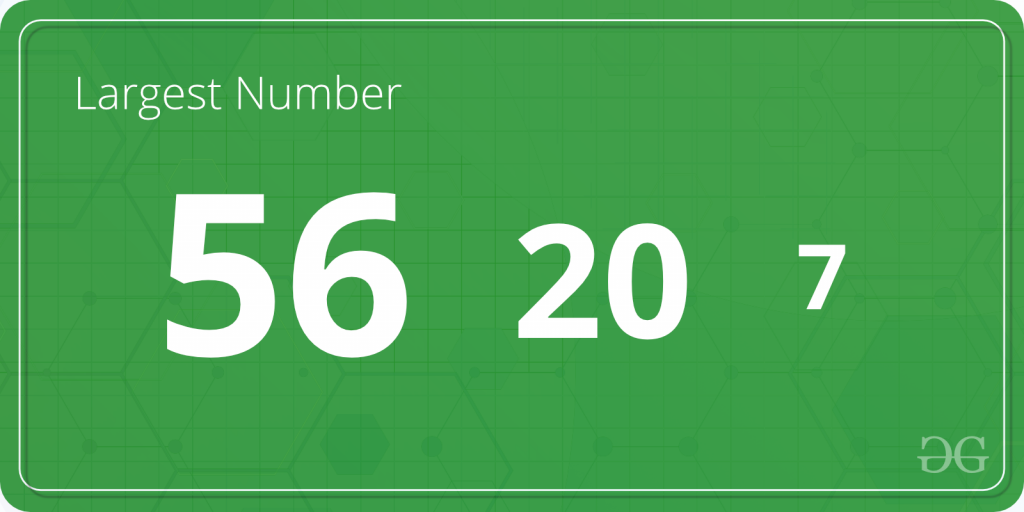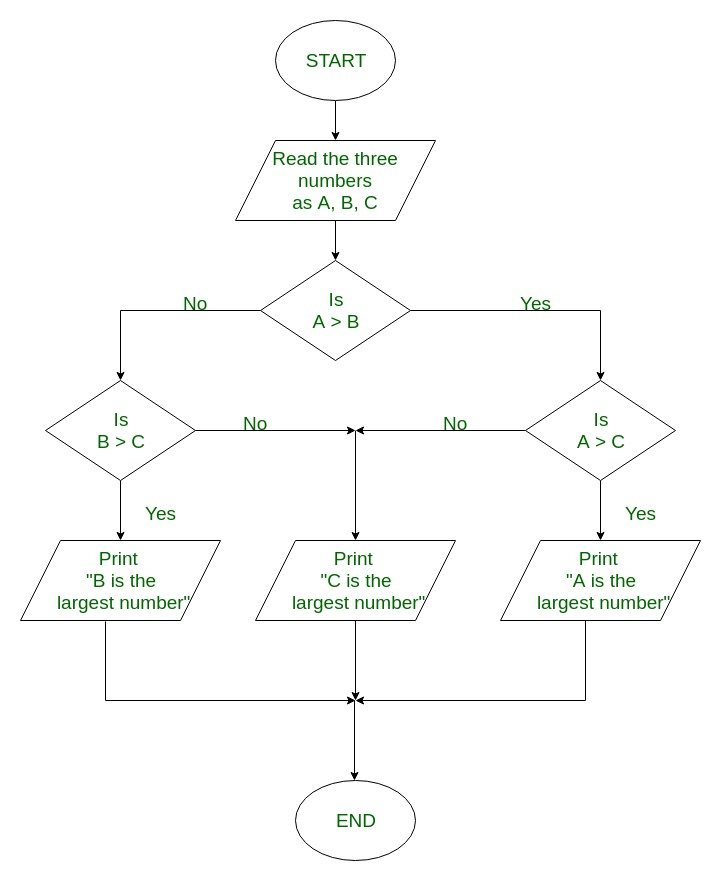# C program to Find the Largest Number Among Three Numbers

• Difficulty Level : Easy
• Last Updated : 26 Dec, 2018

Given three numbers A, B and C; The task is to find the largest number among the three.We provide nothing but the best curated videos and practice problems for our students. Check out the C Foundation Course and master the C language from basic to advanced level. Wait no more, start learning today!

Examples:

```Input: A = 2, B = 8, C = 1
Output: Largest number = 8

Input: A = 231, B = 4751, C = 75821
Output: Largest number = 75821
```

## Recommended: Please try your approach on {IDE} first, before moving on to the solution.

In the below programs, to find the largest of the three number,,,and‘s are used.

Algorithm to find the largest of three numbers:

```1. Start
2. Read the three numbers to be compared, as A, B and C.
3. Check if A is greater than B.

3.1 If true, then check if A is greater than C.
3.1.1 If true, print 'A' as the greatest number.
3.1.2 If false, print 'C' as the greatest number.

3.2 If false, then check if B is greater than C.
3.1.1 If true, print 'B' as the greatest number.
3.1.2 If false, print 'C' as the greatest number.
4. End
```

FlowChart to find the largest of three numbers:Below is the C program to find the largest among the three numbers:

Example 1: Using only if statements to find the largest number.

 `#include `` ` `int` `main()``{``    ``int` `A, B, C;`` ` `    ``printf``(``"Enter the numbers A, B and C: "``);``    ``scanf``(``"%d %d %d"``, &A, &B, &C);`` ` `    ``if` `(A >= B && A >= C)``        ``printf``(``"%d is the largest number."``, A);`` ` `    ``if` `(B >= A && B >= C)``        ``printf``(``"%d is the largest number."``, B);`` ` `    ``if` `(C >= A && C >= B)``        ``printf``(``"%d is the largest number."``, C);`` ` `    ``return` `0;``}`

Output:

```Enter the numbers A, B and C: 2 8 1
8 is the largest number.
```

Example 2: Using if-else statement to find the largest number.

 `#include ``int` `main()``{``    ``int` `A, B, C;`` ` `    ``printf``(``"Enter three numbers: "``);``    ``scanf``(``"%d %d %d"``, &A, &B, &C);`` ` `    ``if` `(A >= B) {``        ``if` `(A >= C)``            ``printf``(``"%d is the largest number."``, A);``        ``else``            ``printf``(``"%d is the largest number."``, C);``    ``}``    ``else` `{``        ``if` `(B >= C)``            ``printf``(``"%d is the largest number."``, B);``        ``else``            ``printf``(``"%d is the largest number."``, C);``    ``}`` ` `    ``return` `0;``}`

Output:

```Enter the numbers A, B and C: 2 8 1
8 is the largest number.
```

Example 3: Using nested if-else statements to find the largest number.

 `#include ``int` `main()``{``    ``int` `A, B, C;`` ` `    ``printf``(``"Enter three numbers: "``);``    ``scanf``(``"%d %d %d"``, &A, &B, &C);`` ` `    ``if` `(A >= B && A >= C)``        ``printf``(``"%d is the largest number."``, A);`` ` `    ``else` `if` `(B >= A && B >= C)``        ``printf``(``"%d is the largest number."``, B);`` ` `    ``else``        ``printf``(``"%d is the largest number."``, C);`` ` `    ``return` `0;``}`

Output:

```Enter the numbers A, B and C: 2 8 1
8 is the largest number.
```

Example 4: Using Ternary operator to find the largest number.

 `#include ``int` `main()``{``    ``int` `A, B, C, largest;`` ` `    ``printf``(``"Enter three numbers: "``);``    ``scanf``(``"%d %d %d"``, &A, &B, &C);`` ` `    ``largest = A > B ? (A > C ? A : C) : (B > C ? B : C);`` ` `    ``printf``(``"%d is the largest number."``, largest);`` ` `    ``return` `0;``}`

Output:

```Enter the numbers A, B and C: 2 8 1
8 is the largest number.
```

My Personal Notes arrow_drop_up